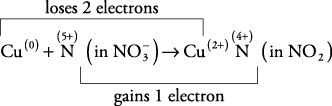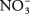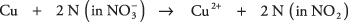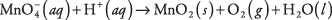## Balancing Equations

A chemical reaction is said to be balanced when the number of atoms of each element is equal in the reactants and products. Because of the conservation of matter, equations are always balanced. You cannot represent the reaction of hydrogen and oxygen as

H 2 + O 2 → H 2O (unbalanced)

because there are more oxygen atoms on the left side. The reaction is correctly written

2H 2 + O 2 → 2H 2O (balanced)

with exactly four hydrogen atoms and two oxygen atoms on each side. The coefficients of the three substances are chosen so the reaction is properly balanced.

Although the brief reactions described to this point may be quickly balanced by inspection or trial‐and‐error, chemistry is rich in complicated reactions that cannot be intuitively balanced. An example is the use of concentrated nitric acid to dissolve copper metal. Here is the unbalanced reaction without any coefficients:Take several minutes and try to balance this equation; the multitude of elements involved makes it a challenging exercise.

Fortunately, such complicated reactions usually involve oxidation and reduction, and the oxidation numbers of each element make it much easier to determine the coefficients for a balanced reaction. First, assign oxidation numbers to the elements in each substance. Examine only the elements that change their oxidation number and insert coefficients so the number of electrons lost equals the number of electrons gained. Then modify any coefficients so the other elements that don't change oxidation number also balance. Finally, check that the electrical charges and the number of atoms of the elements are equal on both sides of the reaction.

As an example, use that method to balance the preceding reaction involving copper and nitric acid. Begin by writing oxidation numbers for all the elements in each substance, as follows:The oxidation number remains constant for hydrogen and oxygen, and you initially can disregard these elements. Only the copper and nitrogen show changing oxidation numbers:The balanced reaction must have equal loss and gain of electrons, so there must be two times as many N atoms as Cu atoms. Insert a coefficient of 2 in front ofand NO 2The electron gain and loss are now balanced:Then placing a coefficient of 4 in front of H+ ( aq) and 2 in front of H 2O ( l) will give the correctly balanced final equation:The preceding technique for balancing reactions is useful because you begin by considering only the elements involved in electron transfer.

• Use oxidation numbers to balance this redox reaction.